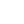# 前言

• Automata theory 研究機械怎樣計算
• Formal language 研究 language, language 是給 automata 計算的東西

# Formal Language

## 一些定義

Language 由 String 的集合 (set)，String 由字母所組成 Language 可以由 Grammar 所產生 (describe) 當每個 grammar 產生的 language 是一樣，那他們就是相同的 grammar

## Regular Language

Regular Language 就是 Finite State Machine 內可以接受 Language Regular expression 是 Regular Language 的 Implementation.

## Context-free grammar (上下文無關連文法)

{% math %} G = (V, T, S, P) {% endmath %} V = variable T = Terminals 最後產生的 terminals S = Starting variable P = set of rules

Context-free grammar 的 rule 可以是 left-recursion or right recursion right recursion 的話可以由 naive recursive parser 進行 parsing, 例子: JSON

PS: recursive parser 是 top down parser

# Automata Theory

## Finite State Machine

### Deterministic finite automaton

state 接受某個字母，只可以轉移到一個 state function 需要是 total function (接受每種可能字母)

### Nondeterministic finite automaton

state 接受某個字母，可以轉移到多於一個 state function 不需要是 total function state 可以接受 empty string (根本是外掛。。)

### NFA = DFA

DNF 不就是 NFA 嘛... 而 NFA 可以轉換為 DNF

** 首先移除 empty string transition** lambda closure: 接受 empty string 可以到達的 state 假設 q0 可以接受 empty string，移除他的 empty string transition

{% math %} \delta'(q0, a) = \lambda-closure( \lambda-closure(q0), a ) {% endmath %}

q0 接受 empty string 到達的 states (set A)，他們接受 a 到那個 states (set B) （很直覺吧... 但不是最後答案) (set B) 再擴展，別忘了它們可以接受 empty string 再走到 (set C) Set C 才是 q0 不用 emtpy string transition 接受 a 可以走到的地方

** 產生 DNF 的 state** 例如 NFA 有三個 state: q0, q1, q2 DNF 最多有的 state 是 q0, q1, q2 的 powerset (2^n) {% math %} \left { \varnothing \right }, \left { q0\right }, \left { q1\right }, \left { q2\right },\left { q0, q1 \right }, \left { q0, q2\right }, \left { q1,q2\right }, \left { q0,q1,q2\right } {% endmath %}

{% math %} \delta(q0, a) = q1 \ \delta(q1, a) = q2 \ \therefore \delta (\left { q0, q1 \right }, a) = \left { q1, q2 \right } {% endmath %}